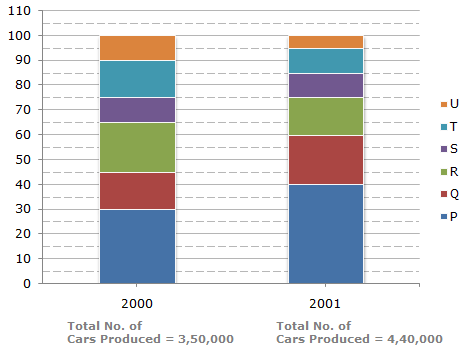# Data Interpretation - Bar Charts

Directions to Solve

The bar graph given below shows the percentage distribution of the total production of a car manufacturing company into various models over two years.

Percentage of Six different types of Cars manufactured by a Company over Two Years1.
What was the difference in the number of Q type cars produced in 2000 and that produced in 2001?
35,500
27,000
22,500
17,500
Explanation:

Total number of Q type cars produced in 2001

=(60 - 40)% of 4,40,000 = 88,000.

Total number of Q type cars produced in 2000

=(45 - 30)% of 3,50,000 = 52,500.Required difference = (88000 - 52500) = 35,500.

2.
Total number of cars of models P, Q and T manufactured in 2000 is?
2,45,000
2,27,500
2,10,000
1,92,500
Explanation:

Analysis of the graph:

We shall first determine the number of cars of each model produced by the Company during the two years:

In 2000 : Total number of cars produced = 3,50,000.

P = (30 - 0)% of 3,50,000 = 30% of 3,50,000 = 1,05,000.

Q = (45 - 30)% of 3,50,000 = 15% of 3,50,000 = 52,500.

R = (65 - 45)% of 3,50,000 = 20% of 3,50,000 = 70,000.

S = (75 - 65)% of 3,50,000 = 10% of 3,50,000 = 35,000.

T = (90 - 75)% of 3,50,000 = 15% of 3,50,000 = 52,500.

U = (100 - 90)% of 3,50,000 = 10% of 3,50,000 = 35,000.

In 2001 : Total number of cars produced = 4,40,000.

P = (40 - 0)% of 4,40,000 = 40% of 4,40,000 = 1,76,000.

Q = (60 - 40)% of 4,40,000 = 20% of 4,40,000 = 88,000.

R = (75 - 60)% of 4,40,000 = 15% of 4,40,000 = 66,000.

S = (85 - 75)% of 4,40,000 = 10% of 4,40,000 = 44,000.

T = (95 - 85)% of 4,40,000 = 10% of 4,40,000 = 44,000.

U = (100 - 95)% of 4,40,000 = 5% of 4,40,000 = 22,000.

Total number of cars of models P, Q and T manufacture in 2000

= (105000 + 52500 + 52500)

= 2,10,000.

3.
If the percentage production of P type cars in 2001 was the same as that in 2000, then the number of P type cars produced in 2001 would have been?
1,40,000
1,32,000
1,17,000
1,05,000
Explanation:

If the percentage production of P type cars in 2001

= Percentage production of P type cars in 2000

= 30%.

then, number of P type cars produced in 2001

= 30% of 4,40,000

= 1,32,000.

4.
If 85% of the S type cars produced in each year were sold by the company, how many S type cars remain unsold?
7650
9350
11,850
12,250
Explanation:

Number of S type cars which remained unsold in 2000 = 15% of 35,000

and number of S type cars which remained unsold in 2001 = 15% of 44,000.Total number of S type cars which remained unsold

= 15% of (35,000 + 44,000)

= 15% of 79,000

= 11,850.

5.
For which model the percentage rise/fall in production from 2000 to 2001 was minimum?
Q
R
S
T
Explanation:

The percentage change (rise/fall) in production from 2000 to 2001 for various models is:

 For P =(176000 - 105000) x 100% = 67.62%, rise. 105000

 For Q =(88000 - 52500) x 100% = 67.62%, rise. 52500

 For R =(70000 - 66000) x 100% = 5.71%, fall. 70000

 For S =(44000 - 35000) x 100% = 25.71%, rise. 35000

 For T =(52500 - 44000) x 100% = 16.19%, fall. 52500

 For U =(35000 - 22000) x 100% = 37.14%, fall. 35000Minimum percentage rise/fall is production is the case of model R.